# Using the Pole Method determine the stresses acting on the horizontal and vertical planes for the...

Using the Pole Method determine the stresses acting on the horizontal and vertical planes for the following: Plane A Normal stress 50 psi compression and Shear Stress 150 psi counter clock wise rotation Plane B Normal stress 250 psi compression and Shear Stress 50 psi clockwise rotation Plane B is oriented 10 degrees counter clockwise from horizontal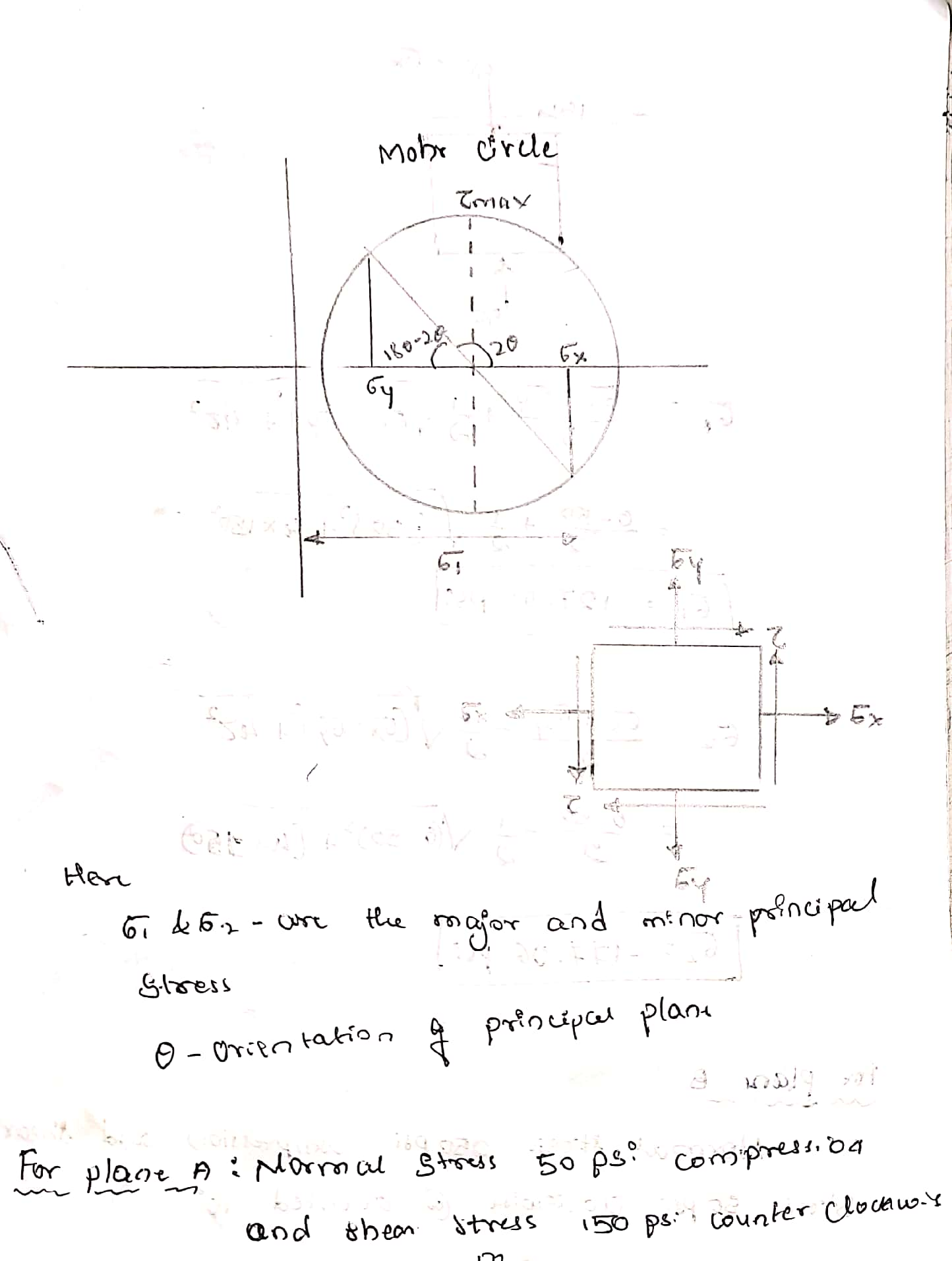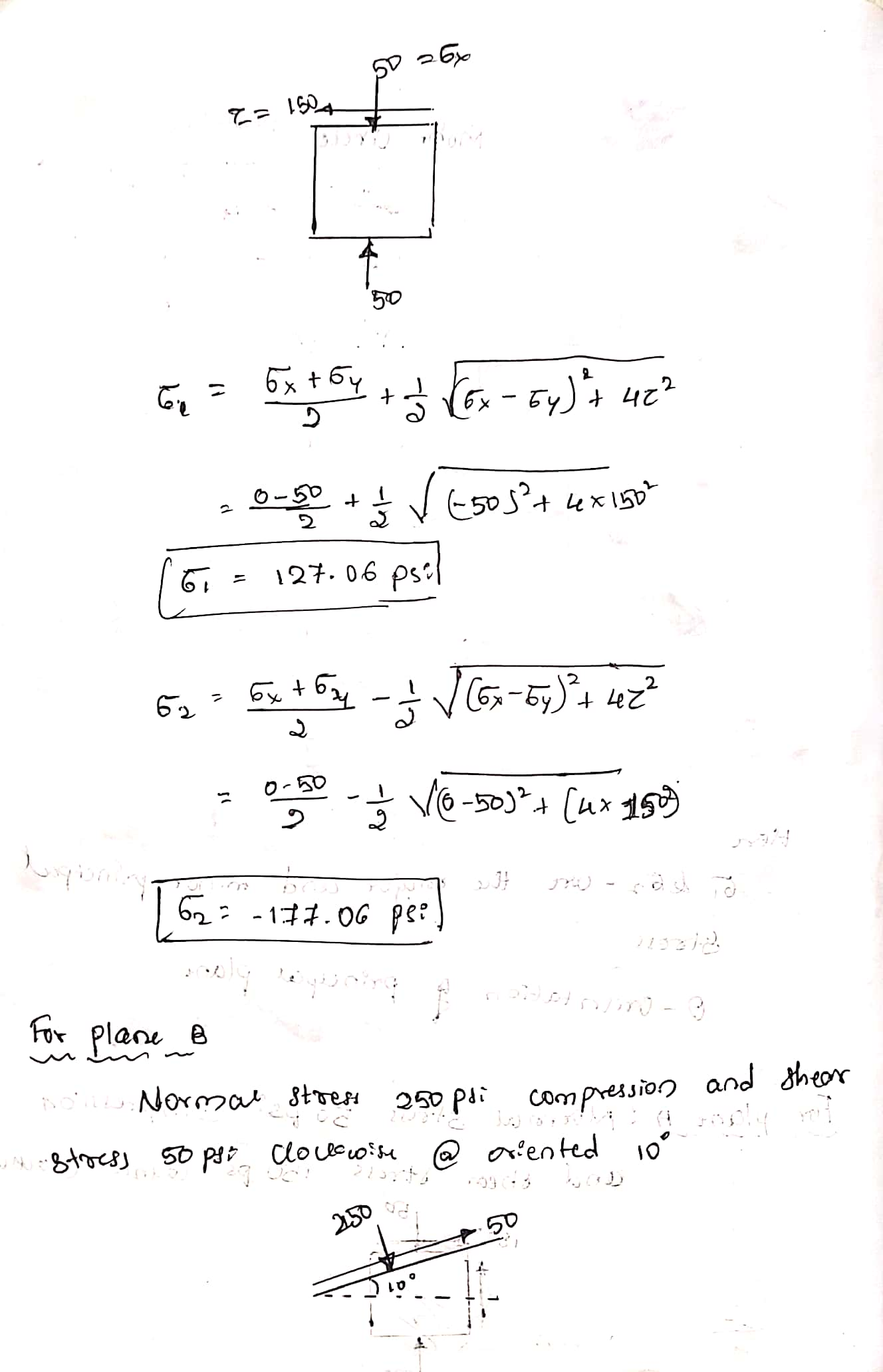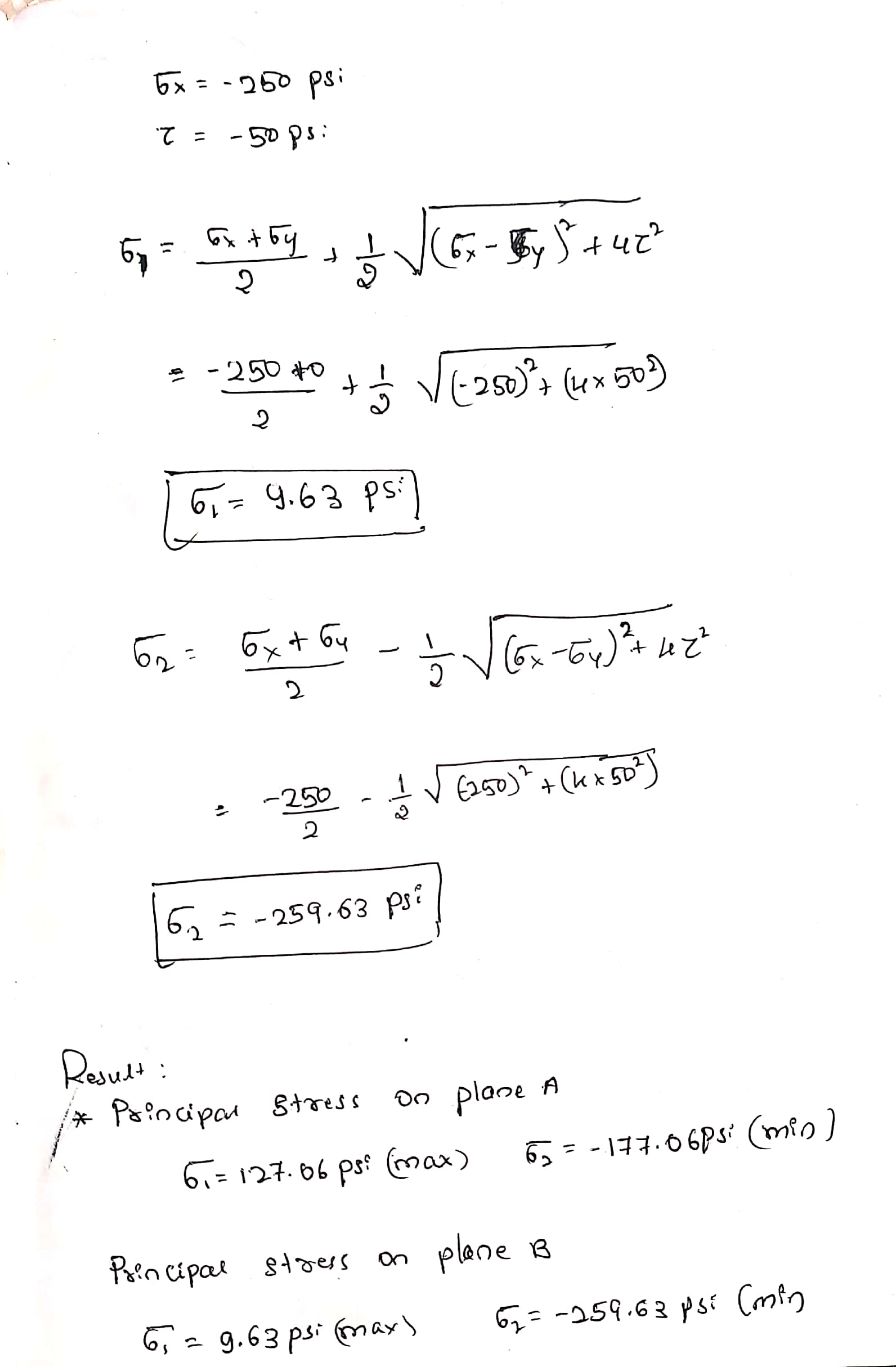#### Earn Coin

Coins can be redeemed for fabulous gifts.

Similar Homework Help Questions
• ### Question 5 Stress Transformation 30 The stresses acting on element A in the web of a...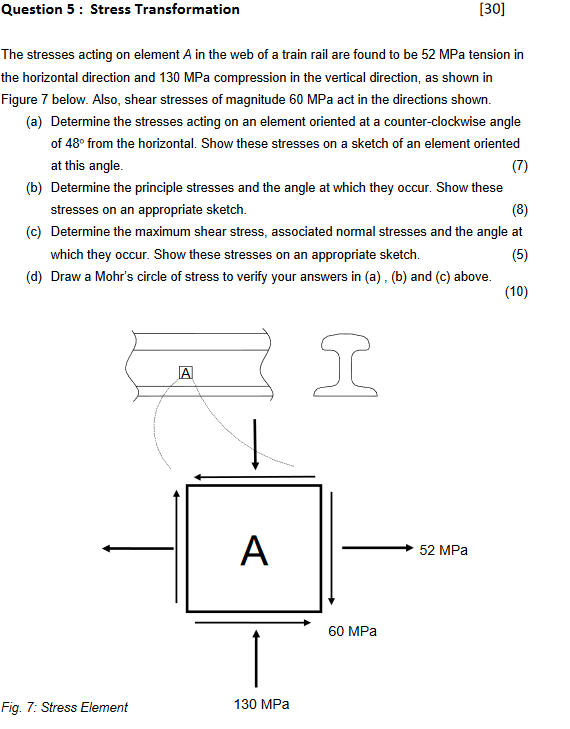Question 5 Stress Transformation 30 The stresses acting on element A in the web of a train rail are found to be 52 MPa tension in the horizontal direction and 130 MPa compression in the vertical direction, as shown in Figure 7 below. Also, shear stresses of magnitude 60 MPa act in the directions shown. (a) Determine the stresses acting on an element oriented at a counter-clockwise angle of 48° from the horizontal. Show these stresses on a sketch of...

• ### EXERCISE 7 (10 PTS) (A) Determine the normal and shear stresses acting on the plane for...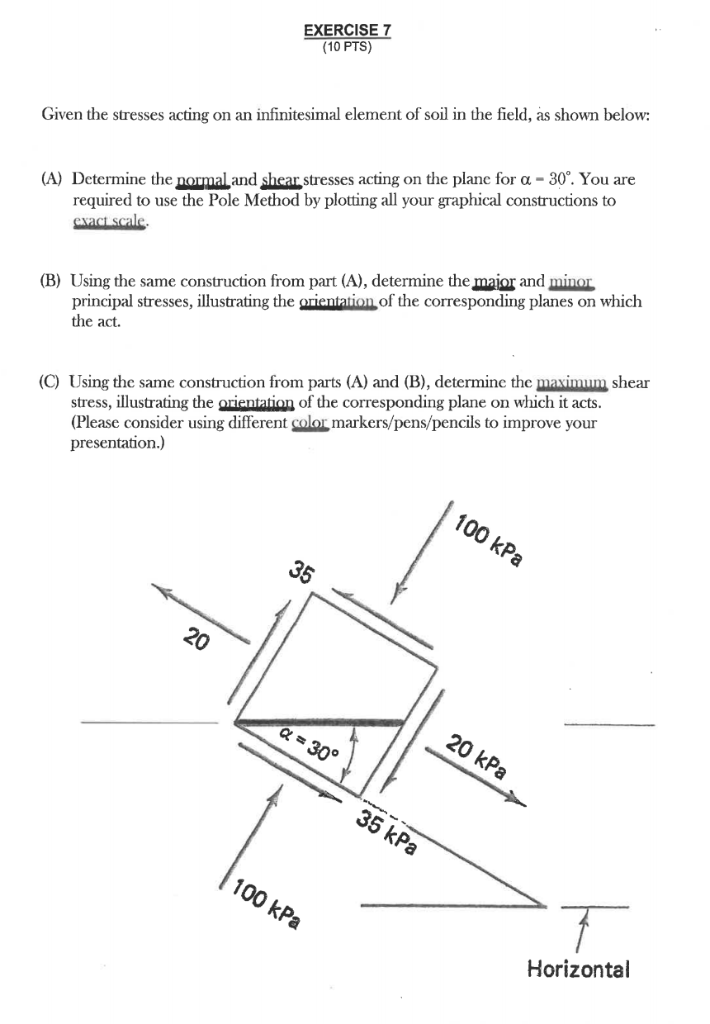EXERCISE 7 (10 PTS) (A) Determine the normal and shear stresses acting on the plane for a - 30°. You are required to use the Pole Method by plotting all your graphical constructions to exact scale Given the stresses acting on an infinitesimal element of soil in the field, as shown below (B) Using the same construction from part (A), determine the major and minor principal stresses, illustrating the orientation of the corresponding planes on which the act (C) Using...

• ### stresses

At a point in a structural member subjected to plane stress, there are the following stresses on horizontal (x) and vertical (y) planes through the point, asfollows:sx = +10,000 psi (i.e., tension)sy = -8,000 psi (i.e., compression)(shear) txy = -4,000 psia) Determine the principal stresses and the maximum shearing stress at the point.b) Locate the planes on which these stresses act and show the stresses on a complete sketch.

• ### Consider a point in a structural member that is subjected to plane stress. Normal and shear...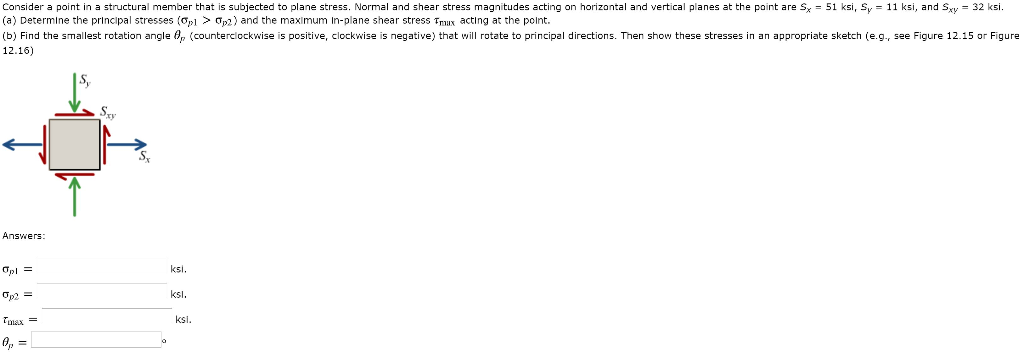Consider a point in a structural member that is subjected to plane stress. Normal and shear stress magnitudes acting on horizontal and vertical planes at the point are Sx = 51 ksi, Sy = 11 ksi, and Sxy = 32 ksi. (a) Determine the principal stresses (01 > 092) and the maximum In-plane shear stress Fruux acting at the point. (b) Find the smallest rotation angle 8, (counterclockwise is positive, clockwise is negative) that will rotate to principal directions. Then...

• ### 2. At a point in a rock layer underground, the vertical and horizontal principal stresses are...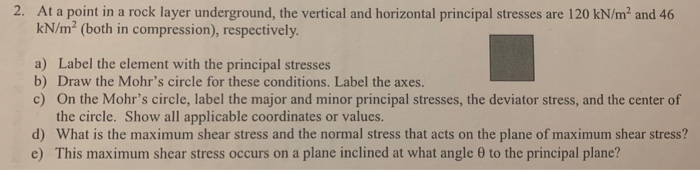2. At a point in a rock layer underground, the vertical and horizontal principal stresses are 120 kN/m2 and 46 kN/m2 (both in compression), respectively. a) Label the element with the principal stresses b) Draw the Mohr's circle for these conditions. Label the axes. c) On the Mohr's circle, label the major and minor principal stresses, the deviator stress, and the center of the circle. Show all applicable coordinates or values d) What is the maximum shear stress and the...

• ### Using Mohr's circle determine for the below differential element: a) the principal stresses and the plan...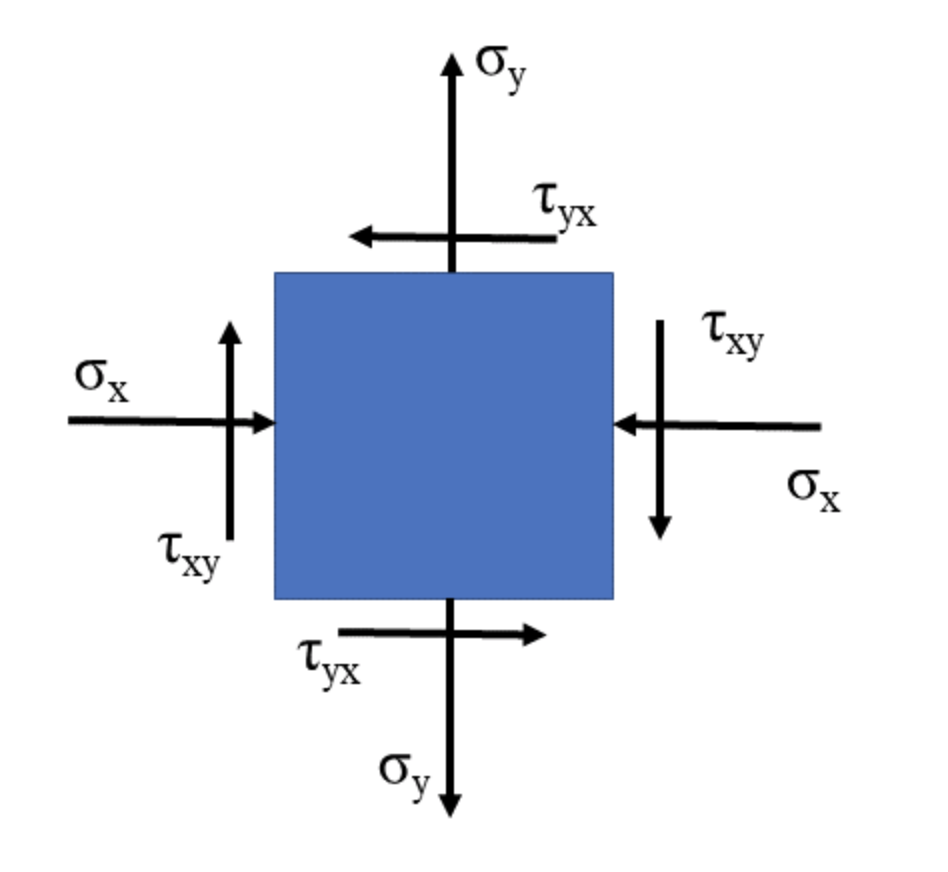Using Mohr's circle determine for the below differential element: a) the principal stresses and the plan on which they act. Show the stresses on a properly oriented differential element. Label all stresses. b) the maximum shear stress and the plan on which they act. Show the stresses on a properly oriented differential element. Label all stresses. c) the stresses on a differential element 40 degrees clockwise from the original element. Show the stresses on a properly oriented differential element. Label...

• ### For the state of stress at a point shown, determine the stresses obtained by rotating the...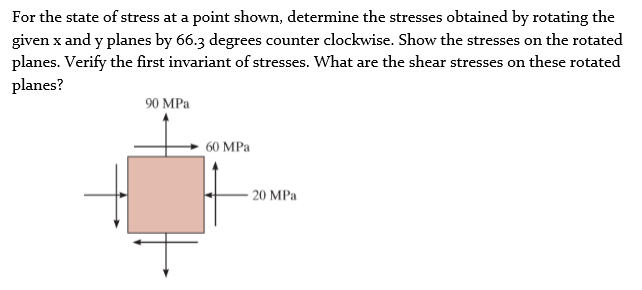For the state of stress at a point shown, determine the stresses obtained by rotating the given x and y planes by 66.3 degrees counter clockwise. Show the stresses on the rotated planes. Verify the first invariant of stresses. What are the shear stresses on these rotated planes? 90 MPa 60 MPa 20 MPa

• ### Consider a point in a structural member that is subjected to plane stress. Normal and shear...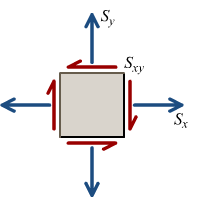Consider a point in a structural member that is subjected to plane stress. Normal and shear stress magnitudes acting on horizontal and vertical planes at the point are Sx = 45 MPa, Sy = 10 MPa, and Sxy = 36 MPa. (a) Determine the principal stresses ( σ p 1 > σ p 2 ) and the maximum in-plane shear stress τ max acting at the point. (b) Find the smallest rotation angle θ p (counterclockwise is positive, clockwise is...

• ### somebody help me plz! : ) P12.043 Consider a point in a structural member that is...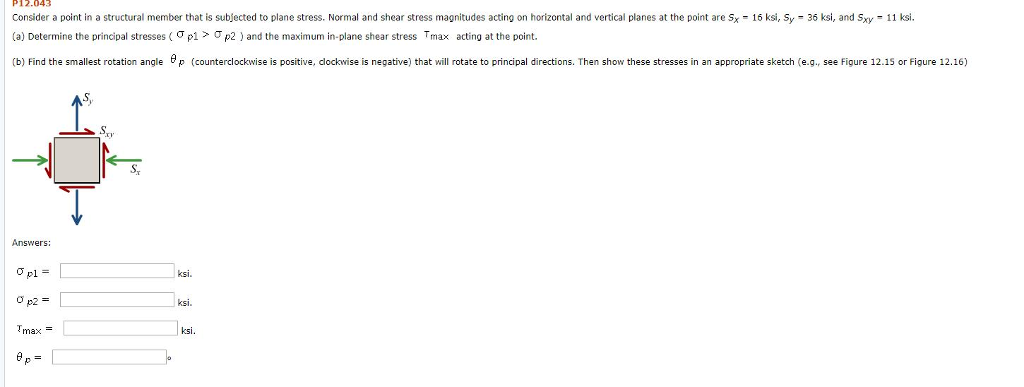somebody help me plz! : ) P12.043 Consider a point in a structural member that is subfected to plane stress. Normal and shear stress magnitudes acting on horizontal and vertical planes at the point are Sx a) Determine the principal stresses ( σ p1 > Ơp2 and the max num in plane shear stress Trax acting at the point. 16 ksi, Sy - 36 ksi, and Sxy 11 ksi (b) Find the smallest rotation agle (counterclockwise is positive, clockwise is...